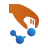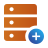# Silicon phonon bandstructure¶

## Introduction¶

In this tutorial you will learn how to perform a simple phonon bandstructure calculation. In particular, you will learn how to set up the calculation and which parameters are critical. You will also learn how to optimize the performance of this type of calculations by an optimal parallelization strategy.

• You will first perform am ATK-DFT calculation with the LDA exchange-correlation potential. You will go through all the relevant parameters used in a phonon calculation.
• Towards the end, you will use the classical potentials available in the ATK-ForceField engine and compare the results.

## Lattice optimization¶

The first, and very important, step is to perform a stress optimization of your system. This removes negative frequencies in the phonon spectrum, which are in most cases due to a non-fully relaxed structure.

1. Go to the Builderand import the Silicon (alpha) structure from the Database.

2. Send the structure to the Script Generatorand add a LCAOCalculator:

• In the Main section, in Basic Parameters select LDA for the Exchange correlation.
• In the k-points box, select Sampling and set it to 13x13x13
• In the Iteration Control section, set a Damping factor of 0.5. This value reduces the number of iterations needed to converge the SCF state for this particular system.
• In the LCAO Basis Set section, set the Pseudopotential to FHI. Then in the Basis set column in the table select SingleZetaPolarized. This is a minimal basis set that is used to speed up the calculations.
3. Add an Optimization ‣ OptimizeGeometry block, and modify the parameters as in the figure below to perform a force and stress minimization.4. Change the output filename to Silicon_opt.hdf5, send the script to the Job Manager, and run it.

The calculation will take just a few seconds. Afterwards, you will find the optimized BulkConfiguration_1 in the LabFloor with the file name Silicon_opt.hdf5. Select this object and click on General Info ‣ Text Representation to get the optimized lattice parameters, 5.4784 Angstrom.## Set up the phonon bandstructure calculation¶

Phonons are calculated from the dynamical matrix of the system. The dynamical matrix is the second derivative of the energy, corresponding to the first derivative of the forces. The first derivative of the forces are calculated using a finite difference scheme, where each symmetrically unique atom in the central cell is displaced along all cartesian directions, also called frozen phonon calculations.

For crystals with small unit cells, a periodically repeated super-cell of the unit cell is often needed to accurately account for long-range interactions in the dynamical matrix. QuantumATK can handle this automatically. By default QuantumATK uses enough repetitions so that each atom is at least 4 covalent bond radii away from its translated copies. This normally includes atoms that are up to 4 bonds away. This is suitable for most systems.

It is also possible to directly set the number of repetitions used in each direction. This is done through the DynamicalMatrix study object. This can especially be important in DFT calculations, which can become quite heavy depending on the number of repetitions.

Drag and drop the optimized BulkConfiguration to the Scripter.

1. The settings from the LCAOCalculator used to optimize the geometry are automatically imported into the new script. The same settings can be used for the bandstructure calculation.

Note

In previous versions the k-points would need to be scaled to take into account the repeated cell used in the calculation of the Dynamical Matrix. This is no longer needed in the current version, as the k-points are now scaled automatically to speed up the calculation and preserve the accuracy of the settings from the previous calculation.

2. Add a PhononBandStructure analysis object.

• Double-click on it and choose 400 points per segment and the G, X, K, G, L Brillouin zone route.
3. The PhononBandstructure analysis object automatically adds a DynamicalMatrix study object to the script.

• In the Dynamical Matrix object set Repetitions to Custom. Set nA, nB and nC to 7. Press OK4. Change the output filename to SiliconPhBS.hdf5.

To test the effect of using different numbers of repetitions, repeat the calculation setting Repetitions to 3x3x3 and 5x5x5.

## Running the phonon bandstructure calculation¶

You are now ready to run your DFT phonon bandstructure calculation. This can be done by sending the script to the Job Manager using the Send To icon.

However, there are a couple of details to consider before running the job:

Parallelization

In QuantumATK, the dynamical matrix is calculated by parallelizing over displacements. The number of displacements is equal to three times the number of atoms (one displacement per each x, y, z directions). For each displacement, two calculations are performed sequentially, corresponding to + and – directions. This means that the optimal performance is obtained by using the same number of MPI processes as the number of displacements.

For this system, six different displacements need to be calculated separately.

System size

The three systems run in this tutorial, 3x3x3, 5x5x5 and 7x7x7 repetitions, consists of 54, 250 and 686 Silicon atoms, respectively. Besides the running time (see below) you should also consider the amount of memory required. A bulk configuration with 686 silicon atoms run with LDA and with a SZP basis set will take about 3 GB of memory. Each displacement, i.e. each MPI process, uses the same amount of memory. This means that in this case (6 MPI processes) if you run on a single machine you will need at least 18 GB of memory!

Timing

The table below reports the total time required to run a phonon bandstructure calculation with the parameters set above. The calculations are run in parallel with 6 MPI processes on a Xeon e5472 3.0GHz machine:

Repetition 3x3x3 5x5x5 7x7x7
Total time 6m21.44s 41m16.41s 3h06m53.15s

## Analyzing the results¶

When the job is done you will find in the LabFloor the PhononBandStructure object. Select it and use the Bandstructure Analyzer tool to display it.The figure below reports results for the 3x3x3, 5x5x5 and 7x7x7 systems defined above.You immediately notice that the 3x3x3 repetition is too small to give even qualitatively good results, while the intermediate size system is already a pretty fine approximation. However, you can also notice that a small difference exists between the acoustic modes near the $$\Gamma$$-point for the 5x5x5 and the 7x7x7 systems.

The dynamical matrix is now stored together with the BulkConfiguration. This means you can read the dynamical matrix and perform another PhononBandStructure calculation (on a different Brillouin path for example) or a PhononDensityOfStates calculation in just a few seconds:The following figures show the phonon bandstructure and DOS, which you can compare to the results reported in Ref. .## ATK-ForceField¶

In ATK 2014 and newer, a large set of classical potentials is included in the ATK-ForceField engine. These potentials are well suited to study vibrational properties of materials, such as the phonon bandstructure of silicon calculated in this tutorial.

Send the silicon bulk configuration to the Scripter. Delete the imported LCAOCalculator and add a ForceFieldCalculator. Now a stress optimization and phonon bandstructure calculation can be set up as explained above, both in the same script.

The calculation will take just a few seconds on a normal desktop machine.In ForceFieldCalculator select the most appropriate potential available for silicon, such as the Tersoff or the Stillinger and Weber potentials.

Note

For QuantumATK-versions older than 2017, the ATK-ForceField calculator can be found under the name ATK-Classical.

Each potential is provided with a literature reference, which you should check to determine if the potential is well suited for your system and for the property you are interested in.Set up the OptimizeGeometry block and the PhononBandstructure analysis object exactly as for the LCAOCalculator case and run the calculation.

The phonon bandstructure of silicon calculated with the Tersoff_si_2005 potential  is reported in the figure below.References

  Giannozzi et al., “Ab initio calculation of phonon dispersions in semiconductors”, Phys. Rev. B 43, 7231 (1991).
  F. H. Stillinger and T. A. Weber, “Computer simulation of local order in condensed phases of silicon”, Phys. Rev. B, 31, 5262 (1985).